# Algebra II : Mode

## Example Questions

← Previous 1 3 4 5

### Example Question #1 : Mode

A class of statistics students scored the following on their last test: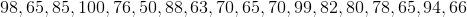.

Calculate the mode for this data set.

Possible Answers: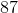Correct answer:Explanation:

The mode is the number that shows up more often than the others in a set of data.

If we put our data set in order we see:

98, 65, 85, 100, 76, 50, 88, 63, 70, 65, 70, 99, 82, 80, 78, 65, 94, 66

Becomes,

50, 63, 65, 65, 65, 66, 70, 70, 76, 78, 80, 82, 85, 88, 94, 98, 99, 100.

Therefore, 65 is the mode because it appears 3 times.

### Example Question #2 : How To Find Mode

What is the mode of the following data set?

{9, 10, 4, 11, 7, 5, 15, 7, 2, 20, 11, 19, 7, 9}

Possible Answers:

7

7 and 9

2

9

11

Correct answer:

7

Explanation:

Arrange the set in numerical order: {2, 4, 5, 7, 7, 7, 9, 9, 10, 11, 11, 15, 19, 20}.

You can then chart the frequency of each value.

Value (Frequency)

2 (1)

4 (1)

5 (1)

7 (3)

9 (2)

10 (1)

11 (2)

15 (1)

19 (1)

20 (1)

From this you can see the most common value, which is the mode, is 7.

### Example Question #2 : How To Find Mode

A set comprises ten elements, which are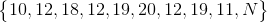wherestands for an unknown number.

What canbe set to in order to make the set bimodal?

Possible Answers:

The set cannot be bimodal for any value of N.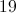Correct answer:Explanation:

12 appears in the set most frequently, appearing three times; 19 is second in frequency, appearing twice. 10, 11, 18, and 20 appear once each.

The only number we can setto to make two data values tie for appearing most frequently is 19; 12 and 19 would then each appear three times, and the others, once. Ifis set to any other number, 12 would remain the most frequent data value.

### Example Question #11 : How To Find Mode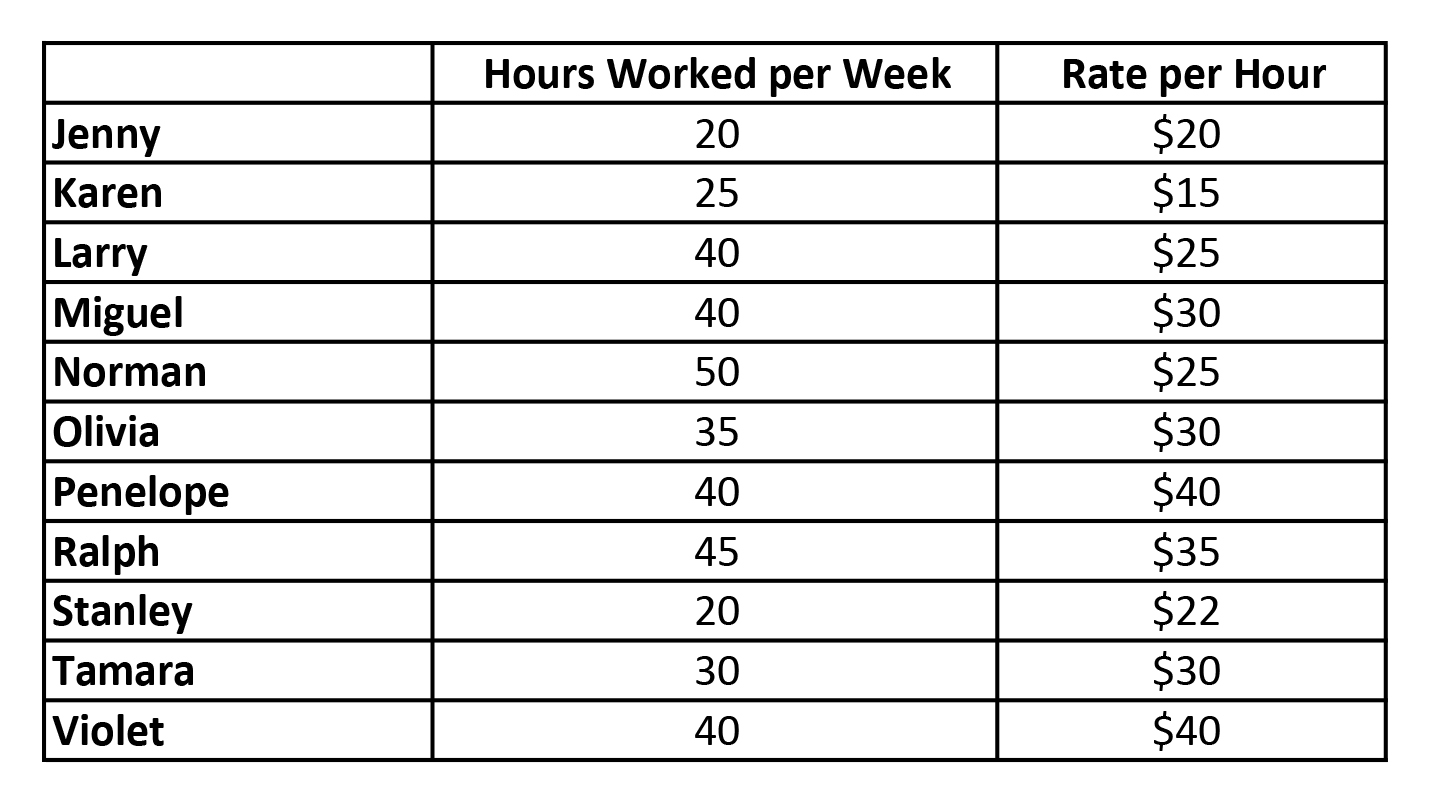Within the set of data of the hours worked per week by the staff, what is the mode?

Possible Answers: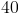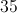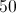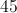Correct answer:Explanation:

Mode is a value that appears most often in a set of data.

In this case, 40 appears 4 times in the set of data, more than any other number.

### Example Question #1 : Mode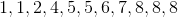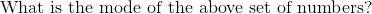Possible Answers:Correct answer:Explanation: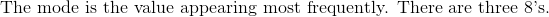### Example Question #6 : Mode

Ms. Anderson's class scored the following on her test: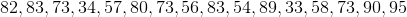What is the mode for this data?

Possible Answers: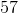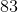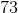Correct answer:Explanation:

The mode is the value that shows up the most often in a set of data.

73 appears 3 times, where no other value appears as often.

Therefore, 73 must be the mode.

### Example Question #7 : Mode

Jason received his scores from the semester. On the last 10 projects, he scored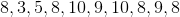(out of 10 points). What is the mode of this data?

Possible Answers: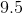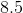Correct answer:Explanation:

The mode is the number that is found most often.

In this case, 8 is found 4 times, 9 is found 2 times, 10 is found 2 times, 3 is found once, and 5 is found once.

Thus, 8 is the mode.

### Example Question #8 : Mode

Find the mode in the data set: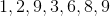Possible Answers:No mode.

Correct answer:Explanation:

The mode is the number that occurs most frequently in the data set.

The only number that is repeated in this data set is.

The correct answer is:### Example Question #9 : Mode

Find the mode: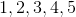Possible Answers:No mode.

Correct answer:

No mode.

Explanation:

The definition of mode in a data set is the most frequent number that occurs in that data set.

Since there is one of each number, there is no number that happens more than once.  Therefore, there is no mode.

The correct answer is:  No mode.

### Example Question #10 : Mode

Find the mode of the following set of numbers: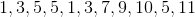.

Possible Answers:Correct answer:Explanation:

The mode of a set is the number that appears most often in the set.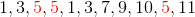In this case, the number 5 appears the most at 3 times.

← Previous 1 3 4 5

### All Algebra II Resources